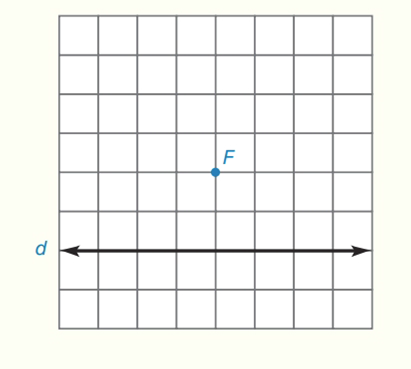Chapter 7.CR, Problem 34CRElementary Geometry For College St...

7th Edition
Alexander + 2 others
ISBN: 9781337614085

Solutions

Chapter
SectionElementary Geometry For College St...

7th Edition
Alexander + 2 others
ISBN: 9781337614085
Textbook Problem

Sketch the parabola that has directrix d and focus F .To determine

To sketch:

The parabola that has directrix d and focus F.

Explanation

Approach:

A parabola is the locus of points that are equidistant t from a fixed line and a fixed point not on that line.

Given:

Draw FAd at point A. Note that FA is the axis of symmetry of the parabola. Locate the midpoint of FA, which is also the vertex V of the parabola.

Through F, draw a line parallel to d. With a compass, measure the distance FA and mark off arcs of that length on the parallel line (on both sides of F)

Still sussing out bartleby?

Check out a sample textbook solution.

See a sample solution

The Solution to Your Study Problems

Bartleby provides explanations to thousands of textbook problems written by our experts, many with advanced degrees!

Get Started

Divide the following fractions and reduce to lowest terms. 22.

Contemporary Mathematics for Business & Consumers

Find the period of y=3cot(x4). 4 8 4

Trigonometry (MindTap Course List)

In Exercises 110, find the graphical solution to each inequality. y3

Finite Mathematics for the Managerial, Life, and Social Sciences

True or False: x2+1x3dx may be solved using a trigonometric substitution.

Study Guide for Stewart's Single Variable Calculus: Early Transcendentals, 8th